Next:Unified Divergence MeasuresUp:Generalized Information Measures

Properties of Unified (r,s)-Inaccuracies

The unified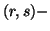inaccuracy measures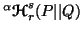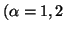and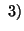satisfy the following properties:

Property 4.13. (Continuity)andare continuous functions of the pair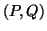and are also continuous with respect to the parameters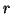and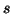.

Property 4.14. (Symmetry)andare symmetric function of their arguments in the pair, i.e.,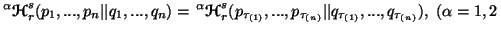and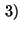where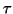is an arbitrary permutation from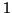to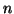.

Property 4.15. (Expansibility) Forand, we have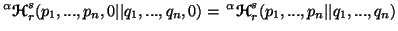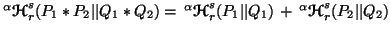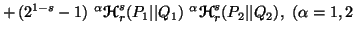andfor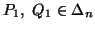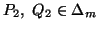and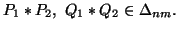Property 4.17. (Nonnegativity)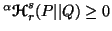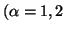andwith equality iff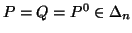, where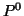is a distribution such that one of the probabilities is one and all others are zero.

Property 4.18. (Monotonicity)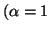and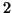) are monotonically increasing functions of(fixed).

Property 4.19. We have

(i)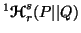is a convex function of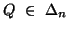for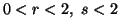and is concave function offor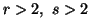.
(ii)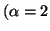andare convex functions offor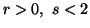.
Property 4.20. (Pseudoconvexity). We have
(i)is pseudoconvex function in Q for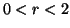and is pseudoconcave in Q for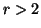.
(ii)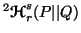is pseudoconvex in Q for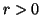.
(iii)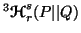is pseudoconvex in Q for.
Note 4.2. It is well known that (ref. Mangasarian, 1969)  every pseudoconvex function is quasiconvex and every pseudoconcave function is quasiconcave. Thus for the respective values of the parametersandin the above results the quasiconvexity (resp. quasiconcavity) follows.

Property 4.21. (Schur-convexity) We have

(i)is Schur-convex function in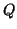for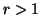and is Schur-concave infor.
(ii)is Schur-convex infor.
(iii)is Schur-convex infor.
Property 4.22. (Shannon-Gibbs-type inequalities) We have
(i)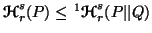, under the following condition: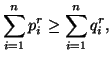(ii)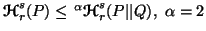and 3.
Note 4.3. The part (i) of the above property can also be proved under the condition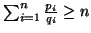, for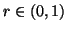using the techniques similar to Kapur (1987) .

Property 4.23. (Inequalities among the measures). For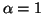and, we have

(i)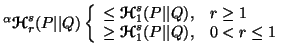(ii)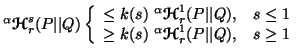where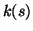is given by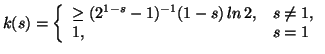(iii)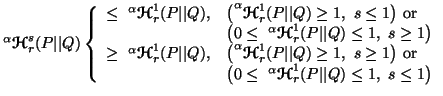for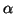=1 and 2.
(iv)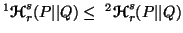, with equality iff either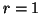or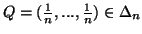.
(v)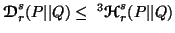,
(vi)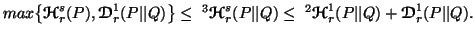(vii)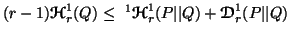.
(viii)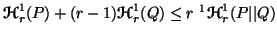.
(ix)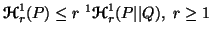.
Note 4.4. The measures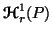and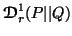are the one parametric generalizations of the Shannon's entropy and Kullback-Leibler's directed divergence respectively studied by Rényi (1961) . The measures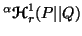(=1,2 and 3) are the three different one parametric generalizations of the Kerridge's (1961)  inaccuracy. The parts (vi) and (vii) connects these measures in an interesting way. But, unfortunately, these are not extendable for the unifiedmeasures. Moreover, the part (vii) don't have sense for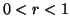, because in this case, the L.H.S. becomes negative. Also for, the inequality (viii) have sense, provided the L.H.S. remains positive.

Note 4.5. Sharma and Mittal (1977)  and Sharma and Gupta (1976)  considered the properties (3.22) and (3.34) along with other postulates to characterize the measures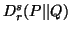and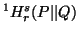respectively. For a simplified characterization ofrefer to Taneja (1984b) .

21-06-2001
Inder Jeet Taneja
Departamento de Matemática - UFSC
88.040-900 Florianópolis, SC - Brazil